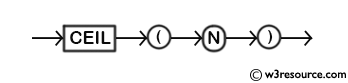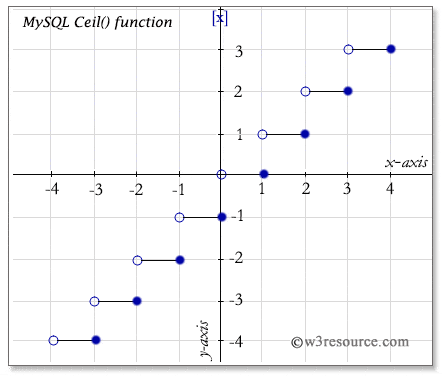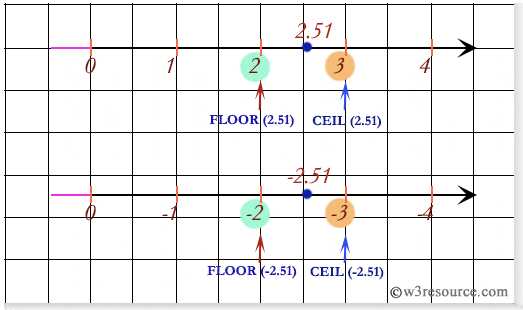# MySQL CEIL() function

## CEIL() function

MySQL CEIL() returns the smallest integer value not less than the number specified as an argument. The synonym of CEIL() is CEILING().

Syntax:

```CEIL(N);
```

Argument :

Name Description
N A number.

Syntax Diagram:MySQL Version: 5.6

Pictorial presentation of CEIL() FunctionExample:

The following MySQL statement returns 3 which is the smallest integer value not less than the value specified (2.2536) in the argument.

Code:

``````SELECT CEIL(2.2536);
```
```

Sample Output:

```mysql> SELECT CEIL(2.2536);
+--------------+
| CEIL(2.2536) |
+--------------+
|            3 |
+--------------+
1 row in set (0.01 sec)
```

Example : CEIL() function with negative value

The following MySQL statement returns -2 which is the smallest integer value not less than the value specified (-2.2536) in the argument.

Code:

``````SELECT CEIL(-2.2536);
```
```

Sample Output:

```mysql> SELECT CEIL(-2.2536);
+---------------+
| CEIL(-2.2536) |
+---------------+
|            -2 |
+---------------+
1 row in set (0.00 sec)
```

Comparing between CEIL() function and FLOOR() functionAll Mathematical FunctionsPrevious: ATAN()
Next: CEILING()

﻿

Inviting useful, relevant, well-written and unique guest posts